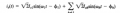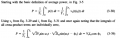# Do current harmonics contribute to active power ?

#### Anestis88

Joined Aug 9, 2011
19
Hi all
While reading Mohan book for power electronics , I am stuck to the statement in the book which says that "current components at harmonic frequencies do not contribute to the average (real) power drawn from the sinusoidal voltage source v "First, I couldn't get it by doing the maths. I can't see how the higher harmonics are eliminated with the "cross products". Also searching in internet I found sites where it is said about "harmonic active power flow" . What is true finally ?

Thanks for any help.

#### Brownout

Joined Jan 10, 2012
2,390
First, I couldn't get it by doing the maths. I can't see how the higher harmonics are eliminated with the "cross products".
Each cross product will have the form Asinwt * Fnsinwnt; there A=sqrt2*Vs as above, Fn is the co-factor of the nth harmonic, and wn is the frequency of the nth harmonic, which is equal to w*N. Thus,

Asinwt * FnsinNw = AFsinwn * sinNwt. The phase term has been left out for simplicity. Focusing only on the sine functions:

Intr{sinwt * sinNwt} = 0 for any whole cycle.

To see why this is, take the integral over one cycle using the identity,

sina * sinb = 1/2[cos (a-b) - cos (a+b) ]

Sorry for my bad symbology. My keyboard is only half working.

Last edited:

Joined Oct 10, 2015
27
In a solely resistive AC circuit, current waveforms are in phase with each other. They change polarity at the same in each cycle. All the power entering the load is dissipated. So, when discussing "real" power, I agree. Harmonics are generated when non-linear 'reactive loads' are introduced.

#### AnalogKid

Joined Aug 1, 2013
10,315
Harmonics are generated when the load impedance changes as a function of the instantaneous value of the input voltage. In other words, a pure inductive load phase shifts the current with respect to the voltage, but does not distort the voltage or current waveforms. For a sinewave source, no distortion, no harmonics. OTOH, (weird example) a resistor tied to a sinewave source through a zener diode conducts during only part of the AC cycle, and the conduction currents for the two half cycles are different. Resistive load + current waveform distortion = no voltage/current phase shift, but lotsa harmonics.

ak

#### Anestis88

Joined Aug 9, 2011
19

#### Brownout

Joined Jan 10, 2012
2,390
Thanks for all the answers, really helped me. Regarding the maths, I finally understand why the higher harmonics equal to zero at the integral of real power. As Brownout said the intergrals [sin(hw)*sin(w)] or [sin(w)*cos(w)] = 0.
BUT in my calculations I still don't get the VsIscosφ because the integral of sin^2 doesn't give zero in a period.
There isn't a sin^2 term in your resulting equation. There is a sinA * sinB. Use the identity given to evaluate it.